If you have a satisfaction question in your survey, or other question where users are asked to rate something on a scale, you can convert this into useful mean values by analysing the responses using a score. You can then have a column that summarises the satisfaction value of all the data.

Scores allow you to assign a value to each code, including a No Response value.

This worksheet shows how to create a score to analyse the satisfaction values in the Crocodile Rock survey supplied with Snap. It explains how to discard the “Don’t know” responses.

### Background

Scores are a way of manipulating your data for analysis. You can assign a score to each question code, and then calculate analyses using the score instead of the code. This is generally used for scoring satisfaction surveys, so that positive ratings are given positive values and negative ratings are given negative values. You can then summarise the whole satisfaction by calculating the mean of all the cases, so you know whether people are generally satisfied or not, by how high or low the mean is.

If people have marked a question as “Don’t Know” or “No opinion” this has different effects on mean values, according to whether it has a value assigned to it or not (even if that value is 0). For example, if you have four respondents to a survey, who have given the following satisfaction values (from a range of -2 to +2).

Person 1: -1

Person 2: +1

Person 3: +2

Person 4: +2

Person 5: Don’t know

To calculate the mean, you sum the values and divide by the number of cases. If you are scoring “Don’t know” as 0, your mean would be (-1 + 1 + 2 + 2 + 0) / 5 or 4/5. If you discard the Don’t Know, your mean would be (-1 + 1 + 2 + 2 )/4 or 4/4. It’s obviously quite important to decide what you do with your “Don’t Know” values in terms of judging the satisfaction of your respondents. By using a score you can choose to score them as a neutral value, or discard them from the calculation.

In the Crocodile Rock survey provided with Snap questions 6 is a satisfaction survey. Respondents are asked to rate how satisfied they are with aspects of the service.

### Step 1: Creating the score weight

The questions to be scored are 6.a to 6.e in the crocodile survey.

1. To check which question this is, open the Crocodile Rock survey (snCrocodile) and scroll down to question 6.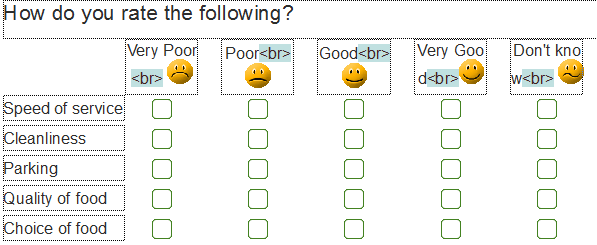2. Click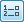or press [Ctrl]+[R] to open the variables window.
3. Find the grid questions and look at the Code list so you know which labels seen by the respondent are assigned to which codes.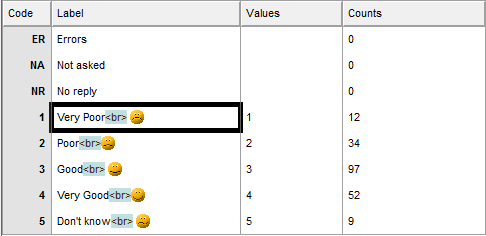4. Open the Weights window by clicking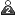or pressing [Ctrl]+[W]. The crocodile survey already has two scores supplied with it.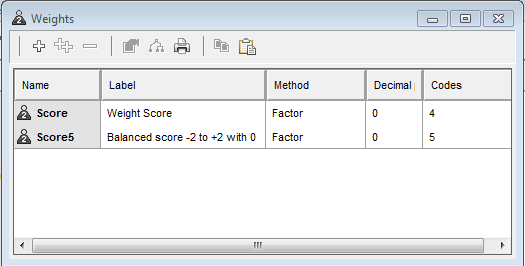5. Click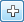to create a new weight. A weight is simply a way of matching values to codes for analysis.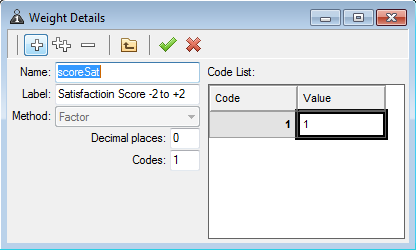6. Name the weight scoreSat so you know what it is. Give it a label explaining what it is used for.
7. Type -2 next to the one in the code list (code 1 is the Very Good value) and click [Tab] to create a new code value. You’ll notice that Snap attempts to guess what value you might want to use.
8. Enter -1 next to code 2. Click [Tab] again.
9. Repeat and enter 1 next to code 3 and 2 next to code 4.
10. Code 5 was the Don’t know response. Type NR to give it a score of no reply. This means that it won’t affect the analysis.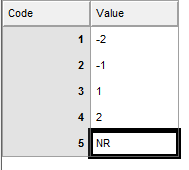Since the Don’t know response was the final one, you could just have omitted code 5 from the weight. In this case, the responses to code 5 would be discarded.

11. Click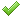to save your score.

### Step 2: Using the score in an analysis

1. Click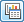to open the Analysis Definition window for a table.
2. Click theto create a new analysis.
3. Set the analysis value to be Q6.a ~Q6.e (this includes all questions from Q.6a to Q6.e).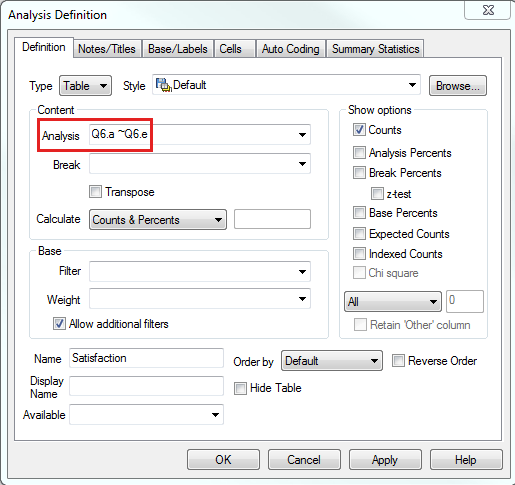4. Leave the type of analysis at Counts and Percents.
5. Name the table, “Satisfaction” in order to identify it easily.
6. Change to the Summary Statistics tab. Type the name of your score (scoreSat) in the Score box. This tells Snap to create a summary of your analysis, scoring the codes with the specified values.7. Click on Mean on the left side in the “Available” column and move it to the “used” column if you want to display the mean.
8. Click [OK] to display the table.
9. There is a column displaying the mean scored values.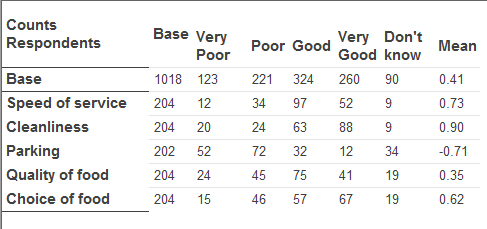The top line (Base) shows the total number of all respondents for all the questions. The mean satisfaction of everyone with all the services is 0.41.The other rows show the average satisfaction for each service. You can see that people are generally positive about the Speed of Service and the Cleanliness and very unhappy about the Parking.

### Step 3: Showing the effect of including and excluding Don’t Know responses

You can examine the effects of including the Don’t Know responses as zeroes by changing the score.

1. Leave the window showing your table open.
2. If the weights window is not already open, clickor press [Ctrl]+[W] to open it.
3. Double-click the scoreSat to open the score window.
4. Edit the last score in the list, changing it from NR to 0.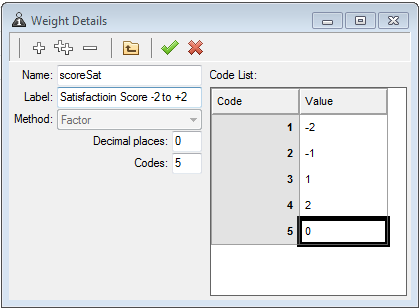5. Click the table window to make it the top window. The Run button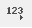will now be in enabled, as the score has been changed since the analysis was performed.
6. Clickor select Edit | Run to recalculate the analysis. The mean values change.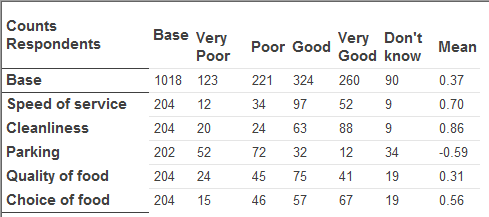7. Clickto save the changes.

If there is a topic you would like a worksheet on, email to snapideas@snapsurveys.com